JEE  >  Test: 35 Year JEE Previous Year Questions: Mechanical Properties of Solids and Fluids

# Test: 35 Year JEE Previous Year Questions: Mechanical Properties of Solids and Fluids

Test Description

## 30 Questions MCQ Test Physics For JEE | Test: 35 Year JEE Previous Year Questions: Mechanical Properties of Solids and Fluids

Test: 35 Year JEE Previous Year Questions: Mechanical Properties of Solids and Fluids for JEE 2022 is part of Physics For JEE preparation. The Test: 35 Year JEE Previous Year Questions: Mechanical Properties of Solids and Fluids questions and answers have been prepared according to the JEE exam syllabus.The Test: 35 Year JEE Previous Year Questions: Mechanical Properties of Solids and Fluids MCQs are made for JEE 2022 Exam. Find important definitions, questions, notes, meanings, examples, exercises, MCQs and online tests for Test: 35 Year JEE Previous Year Questions: Mechanical Properties of Solids and Fluids below.
Solutions of Test: 35 Year JEE Previous Year Questions: Mechanical Properties of Solids and Fluids questions in English are available as part of our Physics For JEE for JEE & Test: 35 Year JEE Previous Year Questions: Mechanical Properties of Solids and Fluids solutions in Hindi for Physics For JEE course. Download more important topics, notes, lectures and mock test series for JEE Exam by signing up for free. Attempt Test: 35 Year JEE Previous Year Questions: Mechanical Properties of Solids and Fluids | 30 questions in 60 minutes | Mock test for JEE preparation | Free important questions MCQ to study Physics For JEE for JEE Exam | Download free PDF with solutions
 1 Crore+ students have signed up on EduRev. Have you?
Test: 35 Year JEE Previous Year Questions: Mechanical Properties of Solids and Fluids - Question 1

### A cylindrical tank has a hole of diameter 2r in its bottom. The hole is covered wooden cylindrical block of diameter 4r, height h and density ρ/3. Situation I : Initially, the tank is filled with water of density ρ to a height such that the height of water above the top of the block is h1 (measured from the top of the block). Situation II : The water is removed from the tank to a height h2 (measured from the bottom of the block), as shown in the figure. The height h2 is smaller than h (height of the block) and thus the block is exposed to the atmosphere.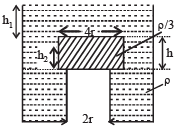Q. Find the minimum value of height h1 (in situation 1), for which the block just starts to move up?

Detailed Solution for Test: 35 Year JEE Previous Year Questions: Mechanical Properties of Solids and Fluids - Question 1

Consider the equilibrium of wooden block.
Forces acting in the downward direction are

(a) Weight of wooden cylinder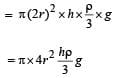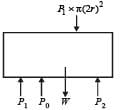(b) Force due to pressure (P1) created by liquid of height h1 above the wooden block is
= P1 × π (2r)2 = [P0 + h1rg] × π (2r)2
Force acting on the upward direction due to pressure P2 exerted from below the wooden block and atmospheric pressure is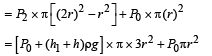At the verge of rising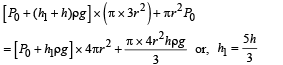Test: 35 Year JEE Previous Year Questions: Mechanical Properties of Solids and Fluids - Question 2

### A cylindrical tank has a hole of diameter 2r in its bottom. The hole is covered wooden cylindrical block of diameter 4r, height h and density ρ/3. Situation I : Initially, the tank is filled with water of density ρ to a height such that the height of water above the top of the block is h1 (measured from the top of the block). Situation II : The water is removed from the tank to a height h2 (measured from the bottom of the block), as shown in the figure. The height h2 is smaller than h (height of the block) and thus the block is exposed to the atmosphere.Q. Find the height of the water level h2 (in situation 2), for which the block remains in its original position without the application of any external force

Detailed Solution for Test: 35 Year JEE Previous Year Questions: Mechanical Properties of Solids and Fluids - Question 2

KEY CONCEPT : Considering equilibrium of wooden block.
Total downward force = Total force upwards Wt. of block + force due to atmospheric pressure = Force due to pressure of liquid + Force due to atmospheric pressure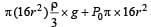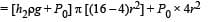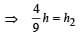Test: 35 Year JEE Previous Year Questions: Mechanical Properties of Solids and Fluids - Question 3

### A cylindrical tank has a hole of diameter 2r in its bottom. The hole is covered wooden cylindrical block of diameter 4r, height h and density ρ/3. Situation I : Initially, the tank is filled with water of density ρ to a height such that the height of water above the top of the block is h1 (measured from the top of the block). Situation II : The water is removed from the tank to a height h2 (measured from the bottom of the block), as shown in the figure. The height h2 is smaller than h (height of the block) and thus the block is exposed to the atmosphere.Q. In situation 2, if h2 is further decreased, then

Detailed Solution for Test: 35 Year JEE Previous Year Questions: Mechanical Properties of Solids and Fluids - Question 3

When the height h2 of water level is further decreased, then the upward force acting on the wooden block decreases. The total force downward remains the same.
This difference will be compensated by the normal reaction by the tank wall on the wooden block. Thus the block does not moves up and remains at its original position.

Test: 35 Year JEE Previous Year Questions: Mechanical Properties of Solids and Fluids - Question 4

When liquid medicine of density ρ is to put in the eye, it is done with the help of a dropper. As the bulb on the top of the dropper is pressed, a drop forms at the opening of the dropper. We wish to estimate the size of the drop. We first assume that the drop formed at the opening is spherical because that requires a minimum increase in its surface energy. To determine the size, we calculate the net vertical force due to the surface tension T when the radius of the drop is R. When this force becomes smaller than the weight of the drop, the drop gets detached from the dropper.

Q. If the radius of the opening of the dropper is r, the vertical force due to the surface tension on the drop of radius R (assuming r << R) is

Detailed Solution for Test: 35 Year JEE Previous Year Questions: Mechanical Properties of Solids and Fluids - Question 4

The vertical force due to surface tension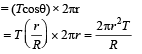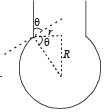Test: 35 Year JEE Previous Year Questions: Mechanical Properties of Solids and Fluids - Question 5

When liquid medicine of density ρ is to put in the eye, it is done with the help of a dropper. As the bulb on the top of the dropper is pressed, a drop forms at the opening of the dropper. We wish to estimate the size of the drop. We first assume that the drop formed at the opening is spherical because that requires a minimum increase in its surface energy. To determine the size, we calculate the net vertical force due to the surface tension T when the radius of the drop is R. When this force becomes smaller than the weight of the drop, the drop gets detached from the dropper.

Q. If r = 5 × 10–4 m, ρ = 103 kgm–3 , g = 10 ms–2 ,T= 0.11Nm –1, the radius of the drop when it detaches from the dropper is approximately

Detailed Solution for Test: 35 Year JEE Previous Year Questions: Mechanical Properties of Solids and Fluids - Question 5

When the drop is about to detach from the dropper Weight = vertical force due to surface tension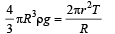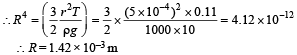Test: 35 Year JEE Previous Year Questions: Mechanical Properties of Solids and Fluids - Question 6

When liquid medicine of density ρ is to put in the eye, it is done with the help of a dropper. As the bulb on the top of the dropper is pressed, a drop forms at the opening of the dropper. We wish to estimate the size of the drop. We first assume that the drop formed at the opening is spherical because that requires a minimum increase in its surface energy. To determine the size, we calculate the net vertical force due to the surface tension T when the radius of the drop is R. When this force becomes smaller than the weight of the drop, the drop gets detached from the dropper.

Q. After the drop detaches, its surface energy is

Detailed Solution for Test: 35 Year JEE Previous Year Questions: Mechanical Properties of Solids and Fluids - Question 6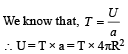Test: 35 Year JEE Previous Year Questions: Mechanical Properties of Solids and Fluids - Question 7

A spray gun is shown in the figure where a piston pushes air out of a nozzle. A thin tube of uniform cross section is connected to the nozzle. The other end of the tube is in a small liquid container.
As the piston pushes air through the nozzle, the liquid from the container rises into the nozzle and is sprayed out. For the spray gun shown, the radii of the piston and the nozzle are 20 mm and 1 mm respectively. The upper end of the container is open to the atmosphere.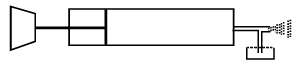Q. If the piston is pushed at a speed of 5 mms–1, the air comes out of the nozzle with a speed of

Detailed Solution for Test: 35 Year JEE Previous Year Questions: Mechanical Properties of Solids and Fluids - Question 7

From principle of continuity,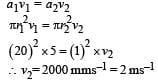Test: 35 Year JEE Previous Year Questions: Mechanical Properties of Solids and Fluids - Question 8

A spray gun is shown in the figure where a piston pushes air out of a nozzle. A thin tube of uniform cross section is connected to the nozzle. The other end of the tube is in a small liquid container.
As the piston pushes air through the nozzle, the liquid from the container rises into the nozzle and is sprayed out. For the spray gun shown, the radii of the piston and the nozzle are 20 mm and 1 mm respectively. The upper end of the container is open to the atmosphere.

Q. If the density of air is ρa, and that of the liquid ρl, then for a given piston speed the rate (volume per unit time) at which the liquid is sprayed will be proportional to

Detailed Solution for Test: 35 Year JEE Previous Year Questions: Mechanical Properties of Solids and Fluids - Question 8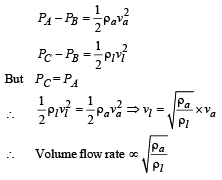Test: 35 Year JEE Previous Year Questions: Mechanical Properties of Solids and Fluids - Question 9

STATEMENT-1 : The stream of water flowing at high speed from a garden hose pipe tends to spread like a fountain when held vertically up, but tends to narrow down when held vertically down.
STATEMENT-2 : In any steady flow of an incompressible fluid, the volume flow rate of the fluid remains constant.

Detailed Solution for Test: 35 Year JEE Previous Year Questions: Mechanical Properties of Solids and Fluids - Question 9

We know that volume flow rate (V) of an incompressible fluid in steady flow remains constant.

V = a × v

where a = area of cross-section and  v =  velocity

⇒ If v decreases a increases and vice - versa.
When stream of water moves up, its speed (v) decreases and therefore 'a' increases i.e. the water spreads out as a fountain. When stream of water from hose pipe moves down, its speed increases and therefore area of crosssection decreases.
Therefore statement-1 is true and statement-2 is the correct explanation of statement-1.

Test: 35 Year JEE Previous Year Questions: Mechanical Properties of Solids and Fluids - Question 10

A spring of force constant 800 N/m has an extension of 5 cm. The work done in extending it from 5 cm to 15 cm is

Detailed Solution for Test: 35 Year JEE Previous Year Questions: Mechanical Properties of Solids and Fluids - Question 10

Small amount of work done in extending the spring by dx is

dW = k x dx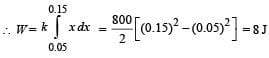Test: 35 Year JEE Previous Year Questions: Mechanical Properties of Solids and Fluids - Question 11

A wire fixed at the upper end stretches by length ℓ by applying a force F. The work done in stretching is

Detailed Solution for Test: 35 Year JEE Previous Year Questions: Mechanical Properties of Solids and Fluids - Question 11

Work done by constant force in displacing the object by a distance ℓ.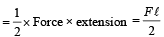Test: 35 Year JEE Previous Year Questions: Mechanical Properties of Solids and Fluids - Question 12

Spherical balls of radius ‘R’ are falling in a viscous fluid of viscosity ‘η’ with a velocity ‘v’. The retarding viscous force acting on the spherical ball is

Detailed Solution for Test: 35 Year JEE Previous Year Questions: Mechanical Properties of Solids and Fluids - Question 12

From Stoke's law,
viscous force F = 6πηrv
hence F is directly proportional to radius & velocity.

Test: 35 Year JEE Previous Year Questions: Mechanical Properties of Solids and Fluids - Question 13

If two soap bubbles of  different radii are connected by a tube.

Detailed Solution for Test: 35 Year JEE Previous Year Questions: Mechanical Properties of Solids and Fluids - Question 13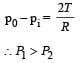hence air moves from smaller bubble to bigger bubble.

Test: 35 Year JEE Previous Year Questions: Mechanical Properties of Solids and Fluids - Question 14

If ‘S’ is stress and ‘Y’ is young’s modulus of material of a wire, the energy stored in the wire per unit volume is

Detailed Solution for Test: 35 Year JEE Previous Year Questions: Mechanical Properties of Solids and Fluids - Question 14

Energy stored per unit volume,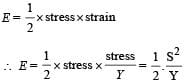Test: 35 Year JEE Previous Year Questions: Mechanical Properties of Solids and Fluids - Question 15

A 20 cm long capillary tube is dipped in water. The water rises up to 8 cm. If the entire arrangement is put in a freely falling elevator the length of water column in the capillary tube will be

Detailed Solution for Test: 35 Year JEE Previous Year Questions: Mechanical Properties of Solids and Fluids - Question 15

Water fills the tube entirely in gravity less condition i.e., 20 cm.

Test: 35 Year JEE Previous Year Questions: Mechanical Properties of Solids and Fluids - Question 16

A wire elongates by l mm when a load W is hanged from it.
If the wire goes over a pulley and two weights W each are hung at the two ends, the elongation of the wire will be (in mm)

Detailed Solution for Test: 35 Year JEE Previous Year Questions: Mechanical Properties of Solids and Fluids - Question 16

Case (i)

At equilibrium, T = W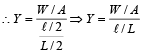Case (ii)   At equilibrium T = W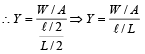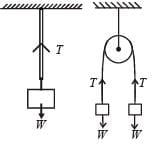⇒ Elongation is the same.

Test: 35 Year JEE Previous Year Questions: Mechanical Properties of Solids and Fluids - Question 17

If the terminal speed of a sphere of gold (density = 19.5 kg/m3) is 0.2 m/s in a viscous liquid (density = 1.5 kg/m3), find the terminal speed of a sphere of silver (density = 10.5 kg/m3) of the same size in the same liquid

Detailed Solution for Test: 35 Year JEE Previous Year Questions: Mechanical Properties of Solids and Fluids - Question 17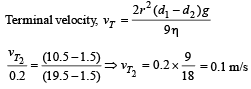Test: 35 Year JEE Previous Year Questions: Mechanical Properties of Solids and Fluids - Question 18

A spherical solid ball of volume V is made of a material of density ρ1. It is falling through a liquid of density ρ22< ρ1). Assume that the liquid applies a viscous force on the ball that is proportional to the square of its speed v, i.e., Fviscous = –kv2 (k > 0). The terminal speed of the ball is

Detailed Solution for Test: 35 Year JEE Previous Year Questions: Mechanical Properties of Solids and Fluids - Question 18

The condition for terminal speed (vt) is Weight = Buoyant force + Viscous force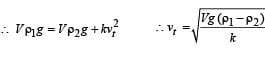Test: 35 Year JEE Previous Year Questions: Mechanical Properties of Solids and Fluids - Question 19

A jar is filled with two non-mixing liquids 1 and 2 having densities ρ1 and, ρ2 respectively. A solid ball, made of a material of density ρ3, is dropped in the jar. It comes to equilibrium in  the position shown in the figure.Which of the following is true for ρ1, ρand ρ3?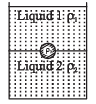Detailed Solution for Test: 35 Year JEE Previous Year Questions: Mechanical Properties of Solids and Fluids - Question 19

From the figure it is clear that liquid 1 floats on liquid  2.
The lighter liquid floats over heavier liquid. Therefore we can conclude that ρ1 < ρ2

Also ρ3 < ρ2 otherwise the ball would have sink to the bottom of the jar.
Also ρ3 > ρ1 otherwise the ball would have floated in liquid 1. From the above discussion we conclude that ρ1 < ρ3 < ρ2.

Test: 35 Year JEE Previous Year Questions: Mechanical Properties of Solids and Fluids - Question 20

A capillary tube (A) is dipped in water. Another identical tube (B) is dipped in a soap-water solution. Which of the following shows the relative nature of the liquid columns in the two tubes?

Detailed Solution for Test: 35 Year JEE Previous Year Questions: Mechanical Properties of Solids and Fluids - Question 20

In case of water, the meniscus sh ape is concave upwards. Also according to ascent formula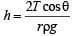The surface tension (T) of  soap solution is less than water. Therefore rise of soap solution in the capillary tube is less as compared to water. As in the case of water, the meniscus shape of soap solution is also concave upwards.

Test: 35 Year JEE Previous Year Questions: Mechanical Properties of Solids and Fluids - Question 21

Two wires are made of the same material and have the same volume. However wire 1 has cross-sectional area A and wire 2 has cross-sectional area 3A. If the length of wire 1 increases by Δx on applying force F, how much force is needed to stretch wire 2 by the same amount?

Detailed Solution for Test: 35 Year JEE Previous Year Questions: Mechanical Properties of Solids and Fluids - Question 21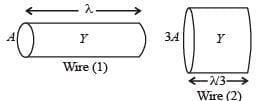As shown in the figure, the wires will have the same Young’s modulus (same material) and the length of the wire of area of cross-section 3A will be  ℓ/3 (same volume as wire 1).
For wire 1,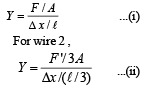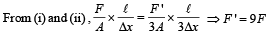Test: 35 Year JEE Previous Year Questions: Mechanical Properties of Solids and Fluids - Question 22

A ball is made of a material of density ρ where ρoil <ρ< ρwater with ρoil and ρwater representing the densities of oil and water, respectively. The oil and water are immiscible. If the above ball is in equilibrium in a mixture of this oil and water, which of the following pictures represents its equilibrium position?

Detailed Solution for Test: 35 Year JEE Previous Year Questions: Mechanical Properties of Solids and Fluids - Question 22

Oil will float on water so, (2) or (4) is the correct option.
But density of ball is more than that of oil,, hence it will sink in oil.

Test: 35 Year JEE Previous Year Questions: Mechanical Properties of Solids and Fluids - Question 23

Two identical charged spheres are suspended by strings of equal lengths. The strings make an angle of 30° with each other. When suspended in a liquid of density 0.8g cm–3, the angle remains the same. If density of the material of the sphere is 1.6 g cm–3 , the dielectric constant of the liquid is

Detailed Solution for Test: 35 Year JEE Previous Year Questions: Mechanical Properties of Solids and Fluids - Question 23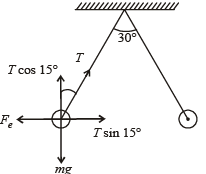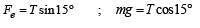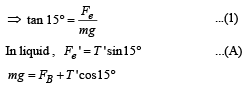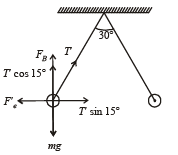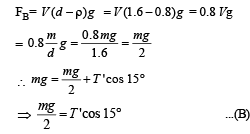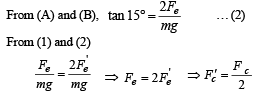Test: 35 Year JEE Previous Year Questions: Mechanical Properties of Solids and Fluids - Question 24

Work done in increasing the size of a soap bubble from a radius of 3 cm to 5 cm is nearly (Surface tension of soap solution = 0.03 Nm–1)

Detailed Solution for Test: 35 Year JEE Previous Year Questions: Mechanical Properties of Solids and Fluids - Question 24

W = T × change in surface area
W = 2T4π[(52) – (3)2] × 10–4
= 2 × 0.03 × 4π [25 – 9] × 10–4 J = 0.4π × 10–3 J
= 0.4π mJ

Test: 35 Year JEE Previous Year Questions: Mechanical Properties of Solids and Fluids - Question 25

Water is flowing continuously from a tap having an internal diameter 8 × 10–3 m. The water velocity as it leaves the tap is 0.4 ms–1. The diameter of the water stream at a distance 2 × 10–1 m below the tap is close to:

Detailed Solution for Test: 35 Year JEE Previous Year Questions: Mechanical Properties of Solids and Fluids - Question 25

From Bernoulli's theorem,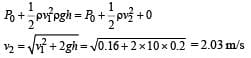From equation of continuity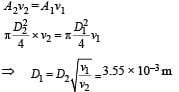Test: 35 Year JEE Previous Year Questions: Mechanical Properties of Solids and Fluids - Question 26

A thin liquid film formed between a U-shaped wire and a light slider supports a weight of 1.5 × 10–2 N  (see figure). The length of the slider is 30 cm and its weight negligible. The surface tension of the liquid film is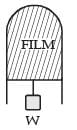Detailed Solution for Test: 35 Year JEE Previous Year Questions: Mechanical Properties of Solids and Fluids - Question 26

At equilibrium,

2Tl = mg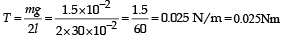Test: 35 Year JEE Previous Year Questions: Mechanical Properties of Solids and Fluids - Question 27

A unifor m cylinder of length L and mass M having crosssectional area A is suspended, with its length vertical, from a fixed point by a massless spring such that it is half submerged in σ liquid of density s at equilibrium position. The extension x0 of the spring when it is in equilibrium is:

Detailed Solution for Test: 35 Year JEE Previous Year Questions: Mechanical Properties of Solids and Fluids - Question 27

From figure,  kx0 + FB = Mg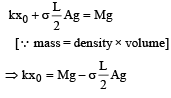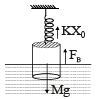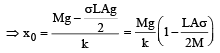Test: 35 Year JEE Previous Year Questions: Mechanical Properties of Solids and Fluids - Question 28

Assume that a drop of liquid evaporates by decrease in its surface energy, so that its temperature remains unchanged.What should be the minimum radius of the drop for this to be possible? The surface tension is T, density of liquid is ρ and L is its latent heat of vaporization.

Detailed Solution for Test: 35 Year JEE Previous Year Questions: Mechanical Properties of Solids and Fluids - Question 28

When radius is decrease by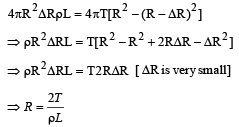Test: 35 Year JEE Previous Year Questions: Mechanical Properties of Solids and Fluids - Question 29

On heating water, bubbles being formed at the bottom of the vessel detach and rise. Take the bubbles to be spheres of radius R and making a circular contact of radius r with the bottom of the vessel. If r << R and the surface tension of water is T, value of r just before bubbles detach is: (density of water is ρ w)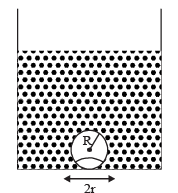Detailed Solution for Test: 35 Year JEE Previous Year Questions: Mechanical Properties of Solids and Fluids - Question 29

(None) None of the given option is correct.
When the bubble gets detached, Buoyant force = force due to surface tension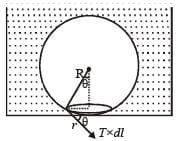Force due to excess pressure =  upthrust Access pressure in air bubble = 2T/R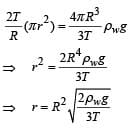Test: 35 Year JEE Previous Year Questions: Mechanical Properties of Solids and Fluids - Question 30

An open glass tube is immersed in mercury in such a way that a length of 8 cm extends above the mercury level. The open end of the tube is then closed and sealed and the tube is raised vertically up by additional 46 cm. What will be length of the air column above mercury in the tube now? (Atmospheric pressure = 76 cm of Hg)

Detailed Solution for Test: 35 Year JEE Previous Year Questions: Mechanical Properties of Solids and Fluids - Question 30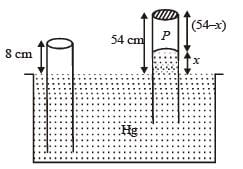Length of the air column above mercury in the tube is, P + x = P0

⇒ P = (76 – x)
⇒ 8 × A × 76 = (76 – x) × A × (54 – x)
∴ x = 38

Thus, length of air column = 54 – 38 = 16 cm.

## Physics For JEE

252 videos|606 docs|257 tests
 Use Code STAYHOME200 and get INR 200 additional OFF Use Coupon Code
Information about Test: 35 Year JEE Previous Year Questions: Mechanical Properties of Solids and Fluids Page
In this test you can find the Exam questions for Test: 35 Year JEE Previous Year Questions: Mechanical Properties of Solids and Fluids solved & explained in the simplest way possible. Besides giving Questions and answers for Test: 35 Year JEE Previous Year Questions: Mechanical Properties of Solids and Fluids, EduRev gives you an ample number of Online tests for practice

## Physics For JEE

252 videos|606 docs|257 tests